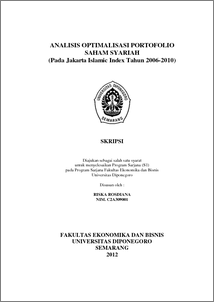# ANALISIS OPTIMALISASI PORTOFOLIO SAHAM SYARIAH (Pada Jakarta Islamic Index Tahun 2006-2010)

ROSDIANA, Riska and SYAICHU, Muhamad (2011) ANALISIS OPTIMALISASI PORTOFOLIO SAHAM SYARIAH (Pada Jakarta Islamic Index Tahun 2006-2010). Undergraduate thesis, Fakultas Ekonomika dan Bisnis.Preview
PDF - Published Version
1343Kb

## Abstract

Portfolio is basically related to how one allocates a number of stocks into various investment types that results on optimal profits. By making diversification, investor may reduce the rate of risk and at the same time optimize the rate of expected return. Based on that, this research raises the problem of how to design an optimal portfolio simulation, i.e. a combination of liquid shares LQ 45 listed in Jakarta Stock Exchange (Now is known as Indonesia Stock Exchange, after the merger with Surabaya Stock Exchange) in the period of 2002-2007 by using Single Index Model and Constant Correlation Model. Single Index Model is a model of portfolio analysis using the account of Excess Return to Beta (ERB) Ratio and value of C* to gain optimal shares on portfolio. The procedure of Constant Correlation Model is exactly parallel to the case of Single Index Model. However, unlike in the Single Index Model, all securities are ranked by Excess Return to Standard Deviation (ERS) instead of Excess Return to Risk. After securities are ranked using the above ratio, securities with greater The hypothesis is there are significant difference between the rate of return and risk optimal portfolio is formed by using a Single Index Model in stock JII in 2006-2010 period. The method of this study is descriptive method. This research take stock sample which covers on index JII for four periods of observation, the amount of stocks samples are 8 stocks sample of portfolio candidates. Technique of collecting data is documentation technique with download data from internet and literature research to get theory base that support the study. Optimal portfolios are formed using Single Index Model gives the average results of portfolio return is 0,1437 and the average portfolio risk is 0,3716. While optimal portfolios are formed using Constant Correlation Model gives the average results of portfolio return is 0,1170 and the average portfolio risk is 0,005712. Based on the results of hypothesis test average difference (t test) showed that the rate of return and risk optimal portfolio is formed by using the Single Index is no different significantly with the rate of return and risk optimal portfolio is formed by using the Constant Correlation. Therefore, the hypothesis is rejected.

Item Type: Thesis (Undergraduate) Optimal Portfolio, Single Index Model, Constant Correlation Model Optimal Portfolio, Single Index Model, Constant Correlation Model H Social Sciences > H Social Sciences (General) Faculty of Economics and Business > Department of Management 35011 Mr. Perpustakaan Fakultas Ekonomi 17 Apr 2012 10:58 17 Apr 2012 10:58

Repository Staff Only: item control page